## √5 and Phi

What is the basis for reason? And mathematics?

Moderators: AMod, iMod

nothing
Posts: 595
Joined: Mon Oct 14, 2019 9:32 pm

### √5 and Phi

(1 + √5) / 2 = 1.618...(Φ)
______________________
1 circle of 360 degrees as r = (1),
+√5 vertices of a pentagram (36x5=180),
/2 possible directions (180x2=360).

(π + π√5) / 2π
yields the same 1.618...(Φ)
and if/when squaring as Φ²
naturally derives the '1'
thus: Φ² = Φ + 1.

π + π√5 / 2π = 1.618... (Φ)
(π + π√5 / 2π)² =
6π² + 2π²√5 / 4π² =
3π + π√5 / 2π = 2.618... Φ + 1
-(π + π√5 / 2π = 1.618... (Φ))
_____________________________
2π, thus π → 3π is '1' full rotation as 2π
or the two "directions" of a pentagram: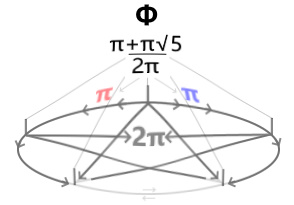By allowing π to produce Φ, the natural emergence of '1'
is the product of their golden arrangement squared, thus
Φ² = Φ + 1 and reflects the bi-directional symmetry
as it exists throughout all of nature, including the human body.

"Opening up" Φ by taking the root of it
yields +/- Φ such to yield the constituency of Φ: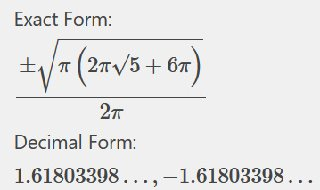____________________________________________
π thus relates to either +/- Φ with 2π capturing both.

1+√5
‾‾ 2 ‾‾ = 1.618...
is thus not as inductively rooted as:
π+π√5
‾ 2π ‾ = 1.618...
which naturally concerns the circle r = 1
if/when squared/reduced:
3π+π√5
‾ 2π ‾ = 2.618...
or simply Φ + 1, a difference of 2π.

Thus, understanding "1" as an qualitative expression of 2π ie. {one full rotation},
lends itself to there being an internal intrinsic symmetry which obeys the "golden rule".
In another manner of speaking: what Φ is to π, Φ² is to 3π, or the "addition" of one full rotation as 2π.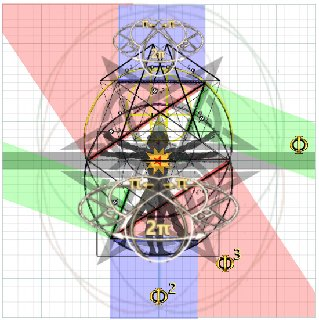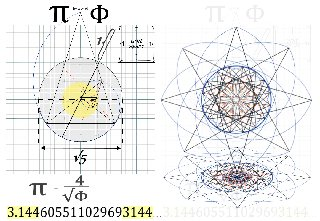Plotting Φ produces a spiral relative to a fixed origin, thus
the arc of it can be used to measure π and found to be
in whole integer relation to Φ. This relationship is presently
ignored by establishment science owing to the same having
established false premises esp. as they concern the nature
(or relative lack thereof) of space and time: they are not
but aspects of motion, thus have no intrinsic geometry
until in relation to one another. The same is true for Φ and π
the former always geometrically satisfying x²-x-1=0 thus
π is only 'transcendental' outside of these parameters
erroneously otherwise taken to be always so.
Last edited by nothing on Wed Mar 18, 2020 10:26 pm, edited 16 times in total.
Sculptor
Posts: 2179
Joined: Wed Jun 26, 2019 11:32 pm

### Re: √5 and Phi

nah
Impenitent
Posts: 2997
Joined: Wed Feb 10, 2010 2:04 pm

### Re: √5 and Phi

Jimmy Walker was almost there...

Phi no mite!

-Imp
wtf
Posts: 969
Joined: Tue Sep 08, 2015 11:36 pm

### Re: √5 and Phi

nothing wrote: Sat Feb 08, 2020 2:42 pm thus
Φ² = Φ + 2π
This cannot be since Φ² - Φ is algebraic and 2π is transcendental.
nothing
Posts: 595
Joined: Mon Oct 14, 2019 9:32 pm

### Re: √5 and Phi

wtf wrote: Sat Feb 08, 2020 9:52 pm
nothing wrote: Sat Feb 08, 2020 2:42 pm thus
Φ² = Φ + 2π
This cannot be since Φ² - Φ is algebraic and 2π is transcendental.
However Φ is a real solution to a quadratic equation.

By allowing use of π to generate both Φ and '1' naturally,
the circle derived/described by Φ² = Φ + 1
(as simply r = 1) is naturally both real and transcendental:
a real circle whose constituency is naturally imbued with Φ
concerning every 2π (ie. r = 1), therefor a coupling of 1:2π
such that golden proportion is the default.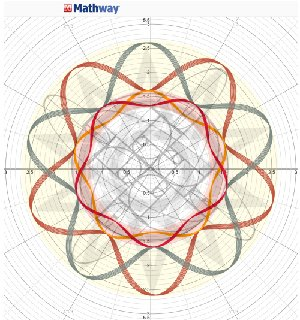Each of the two coils is comprised of two
conjugate pentagrams which all "tap" the circle
at r = 1.618... (Φ) and extends to r = 2.618... (Φ²).
These two coils coincide with the bi-direction symmetry
both: of the pentagram, and of the 2π upon which Φ rests.
Last edited by nothing on Fri Feb 28, 2020 5:46 pm, edited 1 time in total.
wtf
Posts: 969
Joined: Tue Sep 08, 2015 11:36 pm

### Re: √5 and Phi

nothing wrote: Sun Feb 09, 2020 4:53 pm However Φ is a real solution to a quadratic equation.
Yes. That's what makes it algebraic, by definition. And since pi is transcendental -- NOT the solution to any polynomial having integer coefficients -- your equality is false.
Skepdick
Posts: 4964
Joined: Fri Jun 14, 2019 11:16 am

### Re: √5 and Phi

wtf wrote: Sun Feb 09, 2020 8:01 pm Yes. That's what makes it algebraic, by definition. And since pi is transcendental -- NOT the solution to any polynomial having integer coefficients -- your equality is false.
What I am reading into your sentence (by way of implication) is that there is no way to construct a transcendental number from algebraic ones?

Our history aside, I would appreciate a true/false (boolean) response. (only because it's easier than piling on yet more homework).
wtf
Posts: 969
Joined: Tue Sep 08, 2015 11:36 pm

### Re: √5 and Phi

Skepdick wrote: Sun Feb 09, 2020 8:24 pm
wtf wrote: Sun Feb 09, 2020 8:01 pm Yes. That's what makes it algebraic, by definition. And since pi is transcendental -- NOT the solution to any polynomial having integer coefficients -- your equality is false.
What I am reading into your sentence (by way of implication) is that there is no way to construct a transcendental number from algebraic ones?

Our history aside, I would appreciate a true/false (boolean) response. (only because it's easier than piling on yet more homework).
I don't know what you mean by "construct" in this context. If x is transcendental then x - x = 0 is algebraic. So you have to be careful to say exactly what you mean.

OP claimed to have demonstrated the equation Φ² = Φ + 2π. This can not possibly be true. It would imply that Φ² - Φ = 2π. You'd have an algebraic number on the left and a transcendental on the right, being equal. But algebraic and transcendental are mutually exclusive classes of real numbers.

By the way how do I know that Φ² - Φ is algebraic? It's because Φ is algebraic, being the root of a polynomial having integer coefficients, as the OP himself demonstrated. And we know that the product and difference of algebraic numbers is algebraic.

We know that pi is transcendental because Lindemann proved it in 1882.

https://en.wikipedia.org/wiki/Ferdinand ... ence_proof
Skepdick
Posts: 4964
Joined: Fri Jun 14, 2019 11:16 am

### Re: √5 and Phi

wtf wrote: Sun Feb 09, 2020 9:20 pm I don't know what you mean by "construct" in this context. If x is transcendental then x - x = 0 is algebraic. So you have to be careful to say exactly what you mean.
Lets try.

Can I produce some algorithm/computable function which (given infinite time) and using ONLY the set of ALL Algebraic Numbers (if such a set exists? - maybe I am just making it up) as input to this function.

Can such a contraption output a transcendental number?

Although I THINK, you have answered my question by pointing out that x - x = 0. You've given me a fixed point, and I THINK a fixed point between two domains (algebraic and transcendental) is a sufficient criterion for construction.

wtf wrote: Sun Feb 09, 2020 9:20 pm OP claimed to have demonstrated the equation Φ² = Φ + 2π. This can not possibly be true. It would imply that Φ² - Φ = 2π. You'd have an algebraic number on the left and a transcendental on the right, being equal. But algebraic and transcendental are mutually exclusive classes of real numbers.
Yeah, my ask is separate from the OP.
wtf
Posts: 969
Joined: Tue Sep 08, 2015 11:36 pm

### Re: √5 and Phi

Skepdick wrote: Sun Feb 09, 2020 9:24 pm
wtf wrote: Sun Feb 09, 2020 9:20 pm I don't know what you mean by "construct" in this context. If x is transcendental then x - x = 0 is algebraic. So you have to be careful to say exactly what you mean.
Lets try.

Can I produce some algorithm/computable function which (given infinite time) and using ONLY the set of ALL Algebraic Numbers (if such a set exists? - maybe I am just making it up) as input to this function.

Can such a contraption output a transcendental number?
Sure. The sequence of rational (hence algebraic) numbers 3, 3.1, 3.14, 3.141, ... has the limit pi. So an infinite sequence of algebraics can have a transcendental limit. One you allow infinitary processes it's easy to sneak up on transcendentals using only algebraics.

The set of all algebraic numbers exists and is important. It's the set of algebraic numbers. They're a field, meaning you can add, subtract, multiply, and divide (except by 0) without leaving the set.

https://en.wikipedia.org/wiki/Algebraic ... ic_numbers
Skepdick
Posts: 4964
Joined: Fri Jun 14, 2019 11:16 am

### Re: √5 and Phi

wtf wrote: Sun Feb 09, 2020 9:27 pm
Skepdick wrote: Sun Feb 09, 2020 9:24 pm
wtf wrote: Sun Feb 09, 2020 9:20 pm I don't know what you mean by "construct" in this context. If x is transcendental then x - x = 0 is algebraic. So you have to be careful to say exactly what you mean.
Lets try.

Can I produce some algorithm/computable function which (given infinite time) and using ONLY the set of ALL Algebraic Numbers (if such a set exists? - maybe I am just making it up) as input to this function.

Can such a contraption output a transcendental number?
Sure. The sequence of rational (hence algebraic) numbers 3, 3.1, 3.14, 3.141, ... has the limit pi. So an infinite sequence of algebraics can have a transcendental limit. One you allow infinitary processes it's easy to sneak up on transcendentals using only algebraics.
Thanks! I learned something.

Last prod. Is the infinitary process sufficient for getting from algebraic -> transcendental, or is the fixed point (x - x = 0) also necessary?
wtf
Posts: 969
Joined: Tue Sep 08, 2015 11:36 pm

### Re: √5 and Phi

Skepdick wrote: Sun Feb 09, 2020 9:30 pm
Last prod. Is the infinitary process sufficient for getting from algebraic -> transcendental, or is the fixed point (x - x = 0) also necessary?
The algebraic numbers are closed under addition and multiplication (and their inverses). But it's certainly possible for a finite linear combination of transcendental numbers to be algebraic, as the pi - pi = 0 examples shows.

Offhand I can't think of any other classes of examples. But as a striking example of the limits of our knowledge, it is unknown whether e + pi is rational or not. There's no fundamental reason it couldn't be, and nobody's ever proved it one way or the other.

https://math.stackexchange.com/question ... nal-number
Last edited by wtf on Sun Feb 09, 2020 9:36 pm, edited 1 time in total.
Skepdick
Posts: 4964
Joined: Fri Jun 14, 2019 11:16 am

### Re: √5 and Phi

wtf wrote: Sun Feb 09, 2020 9:34 pm The algebraic numbers are closed under addition and multiplication (and their inverses). But it's certainly possible for a finite linear combination of transcendental numbers to be algebraic, as the pi - pi = 0 examples shows. Offhand I can't think of any other classes of examples.
OK. This is harder to parse without some homework.

I'll go take care of that. Thanks!
wtf
Posts: 969
Joined: Tue Sep 08, 2015 11:36 pm

### Re: √5 and Phi

Skepdick wrote: Sun Feb 09, 2020 9:35 pm
wtf wrote: Sun Feb 09, 2020 9:34 pm The algebraic numbers are closed under addition and multiplication (and their inverses). But it's certainly possible for a finite linear combination of transcendental numbers to be algebraic, as the pi - pi = 0 examples shows. Offhand I can't think of any other classes of examples.
OK. This is harder to parse without some homework.

I'll go take care of that. Thanks!
If x is algebraic and y is algebraic then x + y, xy, x - y, and x/y (if y isn't 0) are algebraic. These facts aren't immediately obvious but can be proved.
Skepdick
Posts: 4964
Joined: Fri Jun 14, 2019 11:16 am

### Re: √5 and Phi

wtf wrote: Sun Feb 09, 2020 9:37 pm
Skepdick wrote: Sun Feb 09, 2020 9:35 pm
wtf wrote: Sun Feb 09, 2020 9:34 pm The algebraic numbers are closed under addition and multiplication (and their inverses). But it's certainly possible for a finite linear combination of transcendental numbers to be algebraic, as the pi - pi = 0 examples shows. Offhand I can't think of any other classes of examples.
OK. This is harder to parse without some homework.

I'll go take care of that. Thanks!
If x is algebraic and y is algebraic then x + y, xy, x - y, and x/y (if y isn't 0) are algebraic. These facts aren't immediately obvious but can be proved.
Yeah, that's intuitive to me and I would accept it without proof.

Where I am tripping up is mapping your language to mine so I can grok it e.g "closed under addition...." sounds like what I call total, halting functions etc.